# Time Complexity of Recursive Function

## Time Complexity of Recursive Function

In this article, I am going to discuss How to Find the Time Complexity of a Recursive Function. Please read our previous article, where we discussed How Recursion uses Stack memory in detail.

##### How to Find the Time Complexity of a Recursive Function?

Let us first understand the basic concept for finding the time complexity. We assume that every statement in our program takes one unit of time for execution.

Let me give the idea behind that one. Suppose, there are some books kept in one place and you have to move the book and keep it on a shelf or in a rack. How much time does it take? Maybe half a second, a quarter of a second, maybe if somebody works very slowly may take one second for keeping one book there. The time varies from person to person. So, we don’t mention seconds or milliseconds, we say one unit of time. If you take the example of currency, one dollar, one rupee, one pound. We say one but what is the market value that might be different. So, we say one buck or one unit of currency.

In the same way, we assume that every statement takes one unit of time. If that statement is repeated multiple times, then we need to count the frequency that how many times it is executed. That is sufficient for analyzing our function.

##### Example to Find the Time Complexity of a Recursive Function:

We are going to use the following function. That is, we will calculate the time complexity of the following recursive function.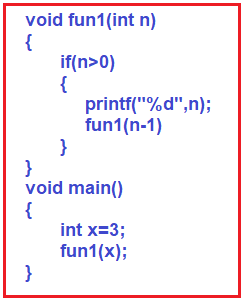Now, let us see what the above function (fun1) is doing. It is doing nothing just printing. It is just printing the value of n.

###### How much time does it take for printing?

It takes one unit of time for printing.

###### How many times the printf is written there?

Only one-time printf is written there. But this is a recursive function. So, it is calling itself again and again.

As it is a recursive function, let us find out how many times the printf function is executed. As we already discussed in our How Recursive Works article, we can find out this using the tracing tree or the recursion tree.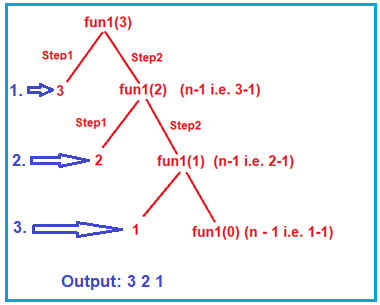As you can see in the above tracing tree, first it prints the value 3, then print 2 and then print the value 1. That means the printf statement executed three times. So, this recursive function will take 3 units of time to execute when the n value is 3. If we make the n value is 5 then it will take 5 units of time to execute this recursive function.

So, we can say for n it will take n units of time. Coming back to the example, if we have to keep one book on a shelf. You will take one unit of time, for 10 books you will take 10 units of time. So, for n number books, you will n unit of time. The most important point that you need to remember is time depends on the number of books.

The time can be represented as the order of n i.e. O(n). The time taken is in order of n.

##### Time Complexity using Recurrence Relation:

There is one more method to find the time complexity i.e. using recurrence relation. Let us see how to write a recurrence relation and how to solve it to find the time complexity of the recursive function. Now, let us find the time complexity of the following recursive function using recurrence relation.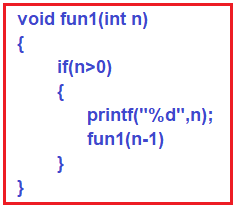We assume that the time taken by the above function is T(n) where T is for time. If the time is taken for fun1() is T(n), then the total time should be the sum of all the times taken by the statements inside that function.

So, let us look at the statement. Every statement takes one unit of time for execution. See there is a conditional statement (if (n > 0)) inside the function. How much time it takes for execution, just one unit of time it takes for execution. Then there is a printf statement, this also takes one unit of time.

Then there is one more function call statement (fun1(n-1)) there, how much time it will take, it also takes one unit of time. No, that is not correct. It will not take one unit of time. This is a function call. It should be the total time taken by that function. It is not just a normal statement. It will call again itself. So, there is something more behind that one. So, we need to know how much time that function call is taking?

Let us see closely. What we said fun1(int n) function call, the total time is T(n). Then this fun1(n-1) is similar to fun1(int n) one, and here it is n-1. So, the total time taken by this function will be T(n-1) time. Then what is T(n)? As we said sum of all the times taken by the statement. So, let us take the sum that is T(n) =T(n-1)+2. For better understanding, please have a look at the below image.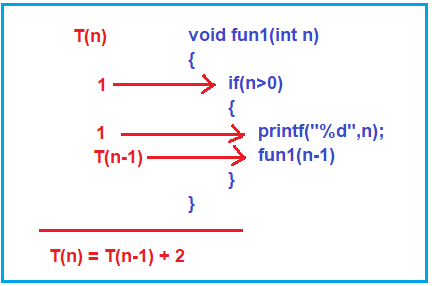So, the recurrence relation is T(n)=T(n-1 )+ 2 when n>0. What happens when n=0, it will just check the condition and it will not enter inside it and will come out. Just checking the condition, so, it will take one unit of time. For better understanding, please have a look at the below image.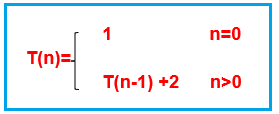So, this is the recurrence formed from that fun1 function. So, the time complexity of the recursive function can be represented in the form of a recurrence relation.

##### Induction Method or Successive Substitution method:

We can also solve this using the induction method also called as successive substitution method and we can get the answer. So, let us solve this one. Before solving this, we should know one thing, if we have any constant value there then we should write it as one 1. In our example, the constant value 2 is there, so replace it with 1 as shown below.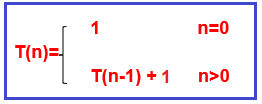So, the recurrence is T(n)=T(n-1) + 1 ———-[eq.1]

We can solve this if we know what is T(n-1)
Since, T(n)=T(n-1) +1
T(n-1) = T(n-2) +1

So, we can substitute T(n-2) +1 in place of T(n-1). So, the next equation is
T(n)=T(n-2) + 1 + 1
T(n) =T(n-2) + 2 ———[eq.2]

Let us substitute T(n-3) +1 in that place then this will be,
T(n)=T(n-3) +1+2
T(n) =T(n-3) +3 ———-[eq.3]

So, we have substituted two times how long we should do this, let us continue it for K times.
T(n)=T(n-k) +k ———[eq.4]

So, go on substituting until it reduces down to a smaller value that is n=0. When we don’t know the answer for a bigger expression, then break the bigger one into a smaller one and solve it. The same thing we have done and we don’t know how much this is, but we know when n=0 then the answer is directly 1. We have tried to reduce this and by substituting and we got that one.

Now, we see that this n-k actually has become 0. Then assume that n-k=0. It means n=k. If we substitute that in [eq.4] it gives,
T(n)=T(n-n) +n
=T(0) +n
=1+n

That solves we got the answer T(n)=1+n. This can be written as O(n). Earlier directly from their tracing tree we also have seen n+1 was the number of calls and the time taken by this fun1 function depends on the number of calls.

In the next article, I am going to discuss Static and Global Variables in Recursion. Here, in this article, I try to explain How to find the time complexity of recursive functions and I hope you enjoy this Time Complexity of a Recursive Function article. Please give your feedback and suggestions about this Time Complexity of a Recursive Function article.